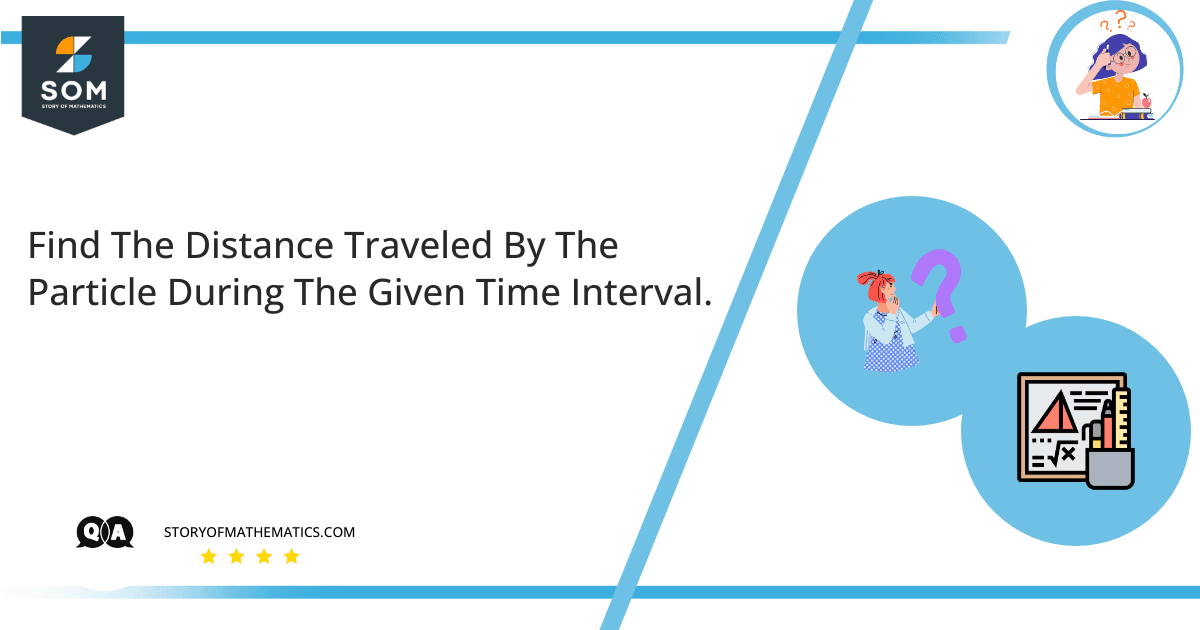# The velocity function (in meters per second) is given for a particle moving along a line.$v(t) = 3t -8, 0 \leq t \leq 3$

(a) Find the displacement.

(b) Find the distance traveled by the particle during the given time interval.

The aim of the question is to understand how to calculate the displacement and the distance covered by the moving particle in the given velocity and the time interval.

Displacement is the change in the position of an object. Displacement is a vector and has direction and magnitude. It is denoted by the arrow that goes from the starting position to the final.

The total distance traveled is calculated by finding the area under the velocity curve from the given time interval.

Part a

Since $v(t) = x'(t)$ where x(t) is the displacement function, then the displacement over the interval $[a,b]$ given $v(t)$ is $\int_a^b v(t) dt$, It is given that $v(t)= 3t-8$ and the interval is $[0,3]$, so the displacement is:

$= \int_0^3 v(t) dt$

$= \int_0^3 (3t-8) dt$

Applying the integration:

$= \left( \dfrac{3} {2} t^2 – 8t \right)_0^3$

Inserting the limits:

$= \left( \dfrac{3} {2} (3)^2 – 8(3) \right) – \left( \dfrac{3} {2} (0)^2 – 8(0) \right)$

$= \dfrac{3} {2} (9) – 24$

$= \dfrac{27} {2} – 24$

$= -10.5$

Part b

Total distance traveled = $\int_a^b |v(t)| dt$ for an interval $[a,b]$. You then determine where $v(t)$ is positive and negative so you can rewrite the integral to have absolute values.

Setting $v(t) = 0$ and solving for $t$ gives:

$0= 3t-8$

$8= 3t$

$t= \dfrac{8} {3}$

Since $t=1$ lies in the interval $[0, \dfrac{8}{3}]$ and $v(t) = 3(1)-8$.

That is $-5$ and $< 0$, then $v(t)<0$ for $[0, \dfrac{8}{3}]$.

Since $t=2.7$ lies in the interval $[\dfrac{8}{3}, 3]$ and $v(t) = 3(2.7)-8$.

That is $0.1$ and $> 0$, then $v(t)>0$ for $[\dfrac{8}{3}, 3]$.

To break apart the absolute value, you then need to write the integral as a sum of integrals over each integral where the interval with $v(t)<0$ has a negative in front and the interval with $v(t)>0$ has a plus front:

$\int_0^3 |v(t)| dt = \int_0^3 |3(t)-8| dt$

$– \int_0^{\dfrac{8} {3}} (3(t)-8) dt + \int_{ \dfrac{8} {3}}^3 (3(t)-8) dt$

$– \left( \dfrac{3}{2} t^2 – 8t \right)_0^{\dfrac{8} {3}} + \left( \dfrac{3}{2} t^2 – 8t \right)_{\dfrac{8} {3}}^3$

$– \left[ \left( \dfrac{3}{2} (\dfrac{8} {3})^2 – 8(\dfrac{8}{3}) \right) – \left( \dfrac{3} {2} (0)^2 – 8(0) \right) \right] + \left[ \left( \dfrac{3}{2} (3)^2 – 8(3) \right) – \left( \dfrac{3} {2} (\dfrac{8}{3})^2 – 8(\dfrac{8} {3}) \right) \right]$

By solving the above expression:

$= \dfrac{32}{3} – \dfrac{21}{2} + \dfrac{32} {3}$

$= \dfrac{65} {6}$

$= 10.833$

Part a: Displacement = $-10.5$

Part b: Distance traveled by the particle is = $10.833$

## Example

Find the displacement if the velocity is given as:

$v(t)= 6- t, 0 \leq t \leq 6$

$= \int_0^6 v(t) dt$

$= \int_0^6 (6-t) dt$

Applying the integration:

$= (6t – \dfrac{1}{2}t^2 )_0^6$

Inserting the limits:

$= (6(6) – \dfrac{1}{2} (6)^2) – ((0)t – \dfrac{1}{2} (0)^2 )$

$= (36 – 18)$

$= 18$